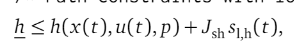# Nonlinear Constraints Problem Formulation

Hello there,

I am currently working on implementing the following two equations as constraints.

In these 2 equations the following is known at runtime and will be given via the parameters: P(k) and P(k-1), lambda.
Phi is a function of x and u, so can be constructed as well.

The first Equation results in a K with the dimensions 40x1. This should be no problem to implement.

The 2nd equation is a Full Matrix tho. Does the acados framework allow this? I tried it with thisbut it seems like h must be a vector.

Is there a standard way to solve this problem? Do it column / row wise?

Thanks in advance and with kind regards,
Albert

Update: Solved via not implementing these as constraints, but its problem specific and not so relevant for other users I think